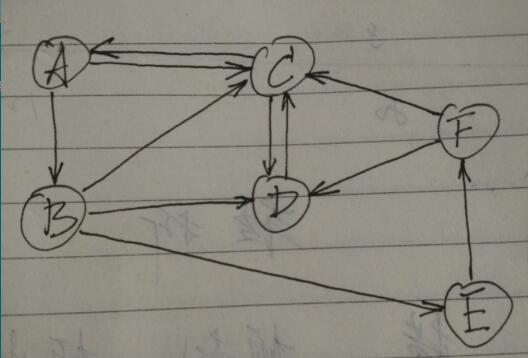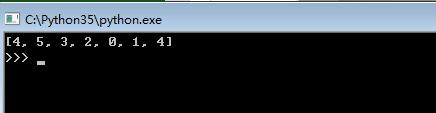# Python基于回溯法子集树模板实现图的遍历功能示例

更新时间：2017年09月05日 11:50:47   作者：罗兵我要评论

A --> B
A --> C
B --> C
B --> D
B --> E
C --> A
C --> D
D --> C
E --> F
F --> C
F --> D```'''

'''
# 用邻接表表示图
n = 6 # 节点数
a,b,c,d,e,f = range(n) # 节点名称
graph = [
{b,c},
{c,d,e},
{a,d},
{c},
{f},
{c,d}
]
x = *(n+1) # 一个解（n+1元数组，长度固定）
X = []     # 一组解
# 冲突检测
def conflict(k):
global n,graph,x
# 第k个节点，是否前面已经走过
if k < n and x[k] in x[:k]:
return True
# 回到出发节点
if k == n and x[k] != x:
return True
return False # 无冲突
# 图的遍历
def dfs(k): # 到达（解x的）第k个节点
global n,a,b,c,d,e,f,graph,x,X
if k > n: # 解的长度超出，已走遍n+1个节点 （若不回到出发节点，则 k==n）
print(x)
#X.append(x[:])
else:
for node in graph[x[k-1]]: # 遍历节点x[k]的邻接节点（x[k]的所有状态）
x[k] = node
if not conflict(k): # 剪枝
dfs(k+1)
# 测试
x = e # 出发节点
dfs(1)  # 开始处理解x中的第2个节点

```